# FREE Step by Step Math Lessons

Our math lessons are designed to make math meaningful to the student. Each math lesson provides in-depth instruction ideal for learners of all ages and abilities. Read the terms and conditions for using our sample lessons below. Get our ad-free, complete math curriculum on our Math Goodies CD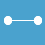## Perimeter and Area of Polygons

Description:

Perimeter of Polygons, Area of Rectangles, Parallelograms, Triangles and Trapezoids. Both metric and English units are used in these math lessons.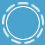## Circumference and Area of Circles

Description:

Geometry, parts of a circle, radius, diameter, Pi, Circumference of Circles, and Area of Circles. Both metric and English units are used in these lessons.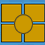## Elementary Math Lessons

Description:

These lessons on number theory include the following topics: Factors, Multiples, Prime and Composite Numbers, Divisibility, Exponents, and Patterns with Exponents. Connections to geometry and the real world.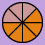## Introduction to Fractions

Description:

Introduction, Classify Fractions, Equivalent Fractions, Simplify, Compare and Order. Convert Fractions to Mixed Numbers. Convert Mixed Numbers to Fractions. Math instruction is visual and conceptual.## Add and Subtract Fractions and Mixed Numbers

Description:

Add and subtract fractions with like and unlike denominators, LCD, add and subtract mixed numbers, solve real-world problems. These lessons use both visual and conceptual approaches.## Multiply and Divide Fractions and Mixed Numbers

Description:

Multiply fractions with and without cancelling, multiply mixed numbers, reciprocals, divide fractions, divide mixed numbers, solving real-world problems. Instruction is visual and conceptual.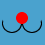Description:

Introduction, Read and Write, Compare and Order Decimals. Estimate Decimal Sums and Differences. Add and Subtract, Solve Decimal Word Problems.## Multiplying and Dividing Decimals

Description:

Estimate Decimal Products and Quotients. Multiply and Divide Decimals by Whole Numbers and by Decimals. Round Decimal Quotients, Solve Word Problems.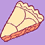## Understanding Percent

Description:

Meaning of Percent, Writing Fractions and Decimals as Percents, Writing Percents as Decimals and Fractions, Percents Less Than 1 or Greater Than 100.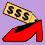## Consumer Math with Percent Applications

Description:

Percent applications are presented, including Percentages and Proportions (IS over OF), Discount, Sale Price, Interest, Commission, Sales Tax, and Percent Change. Many connections to money in these lessons.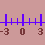## Integer Properties and Arithmetic

Description:

Integer properties and the number line. Absolute Value, Compare, Order, Add, Subtract, Multiply and Divide. Mixed Review of Arithmetic Operations.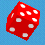## Understanding Probability

Description:

Experiments, Outcomes, Certain and Impossible Events, Sample Spaces, Complement, Mutually Exclusive Events, Addition Rules, Independent and Dependent Events, Conditional Probabilities.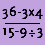## Pre-Algebra

Description:

Order of Operations with Whole Numbers, Integers and Exponents. PEMDAS, Writing Algebraic Expressions and Writing Algebraic Equations.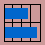## Data and Graphs

Description:

Step-by-step instruction on how to read, interpret and construct Line, Bar and Circle Graphs. Determine which graph is appropriate for a given set of data.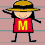## Mean Median Mode

Description:

Data, Range, Mean, Non-Routine Mean Problems, Median, and Mode. Strategies presented for Working Backwards, Bimodal Data, and No Mode.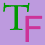## Mathematical Logic

Description:

Negation, Conjunction and Disjunction. Conditional, Compound, and Biconditional Statements. Tautologies and Equivalence. Truth tables used in all lessons.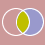## Sets and Set Theory

Description:

Basic definitions and notation, types of sets, equality, Venn diagrams, subsets, Universal set, set-builder notation, complement, intersection and union. Many visuals in these lessons.